## 1 Introduction

Parallel programs and distributed systems become increasingly common. This is driven by the fact that Dennard’s scaling theory , stating that every new processor core is expected to provide a performance gain over older cores, does not hold any more, and instead performance is to be gained from exploiting multiple cores. Consequently, distributed system paradigms such as cloud computing have grown popular. However, designing parallel and distributed systems correctly is notoriously difficult. Unfortunately, it is all too common to observe flaws such as data loss and hanging systems. Although these may be acceptable for many non-critical applications, the occasional hiccup may be impermissible for critical applications, e.g., when giving rise to increased safety risks or financial loss.

The mCRL2 toolset is designed to reason about concurrent and distributed systems. Its language  is based on a rich, ACP-style process algebra and has an axiomatic view on processes. The data theory is rooted in the theory of abstract data types (ADTs). The toolset consists of over sixty tools supporting visualisation, simulation, minimisation and model checking of complex systems.

In this paper, we present an overview of the mCRL2 toolset in general, focussing on the developments from the past six years. We first present a cursory overview of the mCRL2 language, and discuss the recent addition of support for modelling and analysing probabilistic processes.

Behavioural equivalences such as strong and branching bisimulation are used to reduce and compare state spaces of complex systems. Recently, the complexity of branching bisimulation has been significantly improved from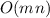to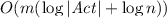, where m is the number of transitions, n the number of states, and $$Act$$ the set of actions. This was achieved by implementing the new algorithm by Groote et al.  . Additionally, support for checking (weak) failures refinement and failures divergence refinement has been added.

Model checking in mCRL2 is based on parameterised boolean equation systems (PBES)  that combine information from a given mCRL2 specification and a property in the modal $$\mu$$-calculus. Solving the PBES answers the encoded model checking problem. Recent developments include improved static analysis of PBESs using liveness analysis, and solving PBESs for infinite-state systems using symbolic quotienting algorithms and abstraction. One of the major features recently introduced is the ability to generate comprehensive counterexamples in the form of a subgraph of the original system.

To aid novice users of mCRL2, an alternative graphical user-interface (GUI), mcrl2ide, has been added, that contains a text editor to create mCRL2 specifications, and provides access to the core functionality of mCRL2 without requiring the user to know the interface of each of the sixty tools. The use of the language and tools is illustrated by means of a selection of case studies conducted with mCRL2. We focus on the application of the tools as a verification back-end for domain specific languages (DSLs), and the verification of software product lines.

The mCRL2 toolset can be downloaded from the website www.mcrl2.org. This includes binaries as well as source code packagesFootnote 1. To promote external contributions, the source code of mCRL2 and the corresponding issue tracker have been moved to GitHub.Footnote 2 The mCRL2 toolset is open source under the permissive Boost license, that allows free use for any purpose. Technical documentation and a user manual of the mCRL2 toolset, including a tutorial, can be found on the website. An extensive introduction to the mCRL2 language can be found in the textbook Modeling and analysis of communicating systems .

The rest of the paper is structured as follows. Section 2 introduces the basics of the mCRL2 language and Sect. 3 its probabilistic extension. In Sect. 4, we discuss several new and improved tools for various behavioural relations. Section 5 gives an overview of novel analysis techniques for PBESs, while Sect. 6 introduces mCRL2’s improved GUI and Sect. 7 discusses a number of applications. Related work is discussed in Sects. 8 and 9 presents a conclusion and future plans.

## 2 The mCRL2 Language and Workflow

The behavioural specification language mCRL2  is the successor of $$\mu$$CRL (micro Common Representation Language ) that was in turn a response to a language called CRL (Common Representation Language) that became so complex that it would not serve a useful purpose.

The languages $$\mu$$CRL and mCRL2 are quite similar combinations of process algebra in the style of ACP  together with equational abstract data types . A typical example illustrating most of the language features of mCRL2 is given in Fig. 1, which shows a filter process ($$Filter$$) that iteratively reads data via an action $$get$$ and forwards it to a queue using the action $$put$$ if the data is not bad. The queue ($$Queue$$) is infinitely sized, reading data via the action $$read$$ and delivering data via the action $$deliver$$. The processes are put in parallel using the parallel operator $$\parallel$$. The actions $$put$$ and $$read$$ are forced to synchronise into the action $$pass\_on$$ using the communication operator $$\mathrm {\Gamma }$$ and the allow operator $$\nabla$$.

The language mCRL2 only contains a minimal set of primitives to express behaviour, but this set is well chosen such that behaviour of communicating systems can be easily expressed. Both $$\mu$$CRL and mCRL2 allow to express systems with time, using positive real time tags to indicate when an action takes place. Recently the possibility has been added to express probabilistic behaviour in mCRL2, which will be explained in Sect. 3.

The differences between $$\mu$$CRL and mCRL2 are minor but significant. In mCRL2 the if-then-else is written as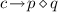(was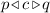). mCRL2 allows for multi-actions, e.g.,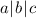expresses that the actions a, b and c happen at the same time. mCRL2 does not allow multiple actions with the same time tag to happen consecutively ($$\mu$$CRL does, as do most other process specification formalisms with time). Finally, mCRL2 has built-in standard datatypes, mechanisms to allow to specify datatypes far more compactly, and it allows for function datatypes, including lambda expressions, as well as arbitrary sets and bags.

The initial purpose of $$\mu$$CRL was to have a mathematical language to model realistic protocols and distributed systems of which the correctness could be proven manually using process algebraic axioms and rules, as well as the equations for the equational data types. The result of this is that mCRL2 is equipped with a nice fundamental theory as well as highly effective proof methods [29, 30], which have been used, for instance, to provide a concise, computer checked proof of the correctness of Tanenbaum’s most complex sliding window protocol .

When the language $$\mu$$CRL began to be used for specifying actual systems , it became obvious that such behavioural specifications are too large to analyse by hand and tools were required, a toolset was developed. It also became clear that specifications of actual systems are hard to give without flaws, and verification is needed to eliminate those flaws. In the early days verification had the form of proving that an implementation and a specification were (branching) bisimilar.

Often it is more convenient to prove properties about aspects of the behaviour. For this purpose mCRL2 was extended with a modal logic, in the form of the modal $$\mu$$-calculus with data and time. A typical example of a formula in modal logic is the following: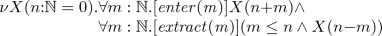which says that the amount extracted using actions $$extract$$ can never exceed the cumulative amount entered via the action $$enter$$. The modal $$\mu$$-calculus with data is far more expressive than languages such as LTL and CTL*, which can be mapped into it .

Verification of modal formulae is performed through transformations to linear process specifications (LPSs) and parameterised boolean equation systems (PBESs) [25, 33]. See Fig. 2 for the typical model checking workflow. An LPS is a process in normal form, where all state behaviour is translated into data parameters. An LPS essentially consists of a set of condition-action-effect rules saying which action can be done in which state, and as such is a symbolic representation of a state space. A PBES is constructed using a modal formula and a linear process. It consists of a parameterised sequence of boolean fixed point equations. A PBES can be solved to obtain an answer to the question whether the mCRL2 specification satisfies the supplied formula. For more details on PBESs and the generation of evidence, refer to Sect. 5.

Whereas an LPS is a symbolic description of the behaviour of a system, a labelled transition system (LTS), makes this behaviour explicit. An LTS can be defined in the context of a set of action labels. The LTS itself consists of a set of states, an initial state, and a transition relation between states where each transition is labelled by an action. The mCRL2 toolset contains the lps2lts tool to obtain the LTS from a given LPS by means of state space exploration. The resulting LTS contains all reachable states of this LPS and the transition relation defining the possible actions in each state. The mCRL2 toolset provides tools for visualising and reducing LTSs and also for comparing LTSs in a pairwise manner. For more details on reducing and comparing LTSs, refer to Sect. 4.

## 3 Probabilistic Extensions to mCRL2

A recent addition to the mCRL2 language is the possibility to specify probabilistic processes using the construct $$\mathbf{dist }~x{:}D[ \, dist (x) \, ].p(x)$$ which behaves as the process p(x) with probability $$dist (x)$$. The distribution $$dist$$ may be discrete or continuous. For example, a process describing a light bulb that fails according to a negative exponential distribution of rate $$\lambda$$ is described as

where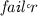is the notation for the action $$fail$$ that takes place at time r.

The modelling of probabilistic behaviour with the probabilistic extension of mCRL2 can be rather insightful as advocated in . There it is illustrated for the Monty Hall problem and the so-called “problem of the lost boarding pass” how strong probabilistic bisimulation and reduction modulo probabilistic weak trace equivalence can be applied to visualise the probabilistic LTS (PLTS) of the underlying probabilistic process as well as to establish the probability of reaching a target state (or set of states). We illustrate this by providing the description and state space of the Monty Hall problem here.

In the Monty Hall problem, there are three doors, one of which is hiding a prize. A player can select a door. Then one of the remaining doors that does not hide the prize is opened. The player can then decide to select the other door. If he does so, he will get the prize with probability $$\frac{2}{3}$$. The action $$\mathtt {prize}( true )$$ indicates that a prize is won. The action $$\mathtt {prize}( false )$$ is an indication that no prize is obtained. A possible model in mCRL2 is given below. In this model the player switches doors. So, the prize is won if the initially selected door was not the door with the prize.

The generated state space for this model is given in Fig. 3 at the left. From probabilistic mCRL2 processes probabilistic transition systems can be generated, which can be reduced modulo strong probabilistic bisimulation  (see the next section). The reduced transition system is provided at the right, and clearly shows that the prize is won with probability $$\frac{2}{3}$$.

Moreover, modal mu-calculus formulae yielding a probability, i.e.  a real number, can be evaluated invoking probabilistic counterparts of the central tools in the toolset. For the Monty Hall model the modal formula $$\langle \mathtt {prize}( true )\rangle true$$ will evaluate to the probability $$\frac{2}{3}$$. The tool that verified this modal formula is presented in . Although the initial results are promising, the semantic and axiomatic underpinning of the process theory for probabilities is demanding.

## 4 Behavioural Relations

Given two LTSs, the ltscompare tool can check whether they are related according to one of a number of equivalence and refinement relations. Additionally, the ltsconvert tool can reduce a given LTS modulo an equivalence relation. In the following subsections the recently added implementations of several equivalence and refinement relations are described.

### 4.1 Equivalences

The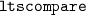tool can check simulation equivalence, and (weak) trace equivalence between LTSs. In the latest release an algorithm for checking ready simulation was implemented and integrated into the toolset . Regarding bisimulations, the tool can furthermore check strong, branching and weak bisimulation between LTSs. The latter two are sensitive to so-called internal behaviour, represented by the action $$\tau$$. Divergence-preserving variants of these bisimulations are supported, which take the ability to perform infinite sequences of internal behaviour into account. The above mentioned equivalences can also be used by the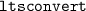tool.

Recently, the Groote/Jansen/Keiren/Wijs algorithm (GJKW) for branching bisimulation , with complexity $$O(m(\log | Act |+\log n))$$, was implemented. When tested in practice, it frequently demonstrates performance improvements by a factor of 10, and occasionally by a factor of 100 over the previous algorithm by Groote and Vaandrager .

The improved complexity is the result of combining the process the smaller half principle  with the key observations made by Groote and Vaandrager regarding internal transitions . GJKW uses partition refinement to identify all classes of equivalent states. Repeatedly, one class (or block) B is selected to be the so-called splitter, and each block $$B'$$ is checked for the reachability of B, where internal behaviour should be skipped over. In case B is reachable from some states in $$B'$$ but not from others, $$B'$$ needs to be split into two subblocks, separating the states from which B can and cannot be reached. Whenever a fixed-point is reached, the obtained partition defines the equivalence relation.

GJKW applies process the smaller half in two ways. First of all, it is ensured that each time a state s is part of a splitter B, the size of B, in terms of number of states, is at most half the size of the previous splitter in which s resided. To do this, blocks are partitioned in constellations. A block is selected as splitter iff its size is at most half the number of states in the constellation in which it resides. When a splitter is selected, it is moved into its own, new, constellation, and when a block is split, the resulting subblocks remain in the same constellation.

Second of all, it has to be ensured that splitting a block $$B'$$ takes time proportional to the smallest resulting subblock. To achieve this, two state selection procedures are executed in lockstep, one identifying the states in $$B'$$ that can reach the splitter, and one detecting the other states. Once one of these procedures has identified all its states, those states can be split off from $$B'$$.

Reachability checking is performed efficiently by using the notion of bottom state , which is a state that has no outgoing internal transitions leading to a state in the same block. It suffices to check whether any bottom state in $$B'$$ can reach B. Hence, it is crucial that for each block, the set of bottom states is maintained during execution of the algorithm.

GJKW is very complicated due to the amount of book keeping needed to achieve the complexity. Among others, a data structure by Valmari, called refinable partition  is used, together with three copies of all transitions, structured in different ways to allow fast retrieval in the various stages of the algorithm.

Besides checking for branching bisimulation, GJKW is used as a basis for checking strong bisimulation (in which case it corresponds to the Paige-Tarjan algorithm ) and as a preprocessing step for checking weak bisimulation.

For the support of the analysis of probabilistic systems, a number of preliminary extensions have been made to the mCRL2 toolset. In particular, a new algorithm has been added to reduce PLTSs – containing both non-deterministic and probabilistic choice  – modulo strong probabilistic bisimulation. This new Paige-Tarjan style algorithm, called GRV  and implemented in the tool ltspbisim, improves upon the complexity of the best known algorithm so far by Baier et al.  . The GRV algorithm was inspired by work on lumping of Markov Chains by Valmari and Franceschinis  to limit the number of times a probabilistic transition needs to be sorted. Under the assumption of a bounded fan-out for probabilistic states, the time complexity of GRV is $$O( n_p \log n_a)$$ with $$n_p$$ equal to the number of probabilistic transitions and $$n_a$$ being the number of non-deterministic states in a PLTS.

### 4.2 Refinement

In model checking there is typically a single model on which properties, defined in another language, are verified. An alternative approach that can be employed is refinement checking. Here, the correctness of the model is verified by establishing a refinement relation between an implementation LTS and a specification LTS. The chosen refinement relation must be strong enough to preserve the desired properties of the model, but also weak enough to allow many valid implementations.

For refinement relations the ltscompare tool can check the asymmetric variants of simulation, ready simulation and (weak) trace equivalence between LTSs. In the latest release, several algorithms have been added to check (weak) trace, (weak) failures and failures-divergences refinement relations based on the algorithms introduced in . We remark that weak failures refinement is known as stable failures refinement in the literature. Several improvements have been made to the reference algorithms and the resulting implementation has been successfully used in practice, as described in Sect. 7.1.

The newly introduced algorithms are based on the notion of antichains. These algorithms try to find a witness to show that no refinement relation exists. The antichain data structure keeps track of the explored part of the state space and assists in pruning other parts based on an ordering. If no refinement relation exists, the tool provides a counterexample trace to a violating state. To further speed up refinement checking, the tool applies divergence-preserving branching bisimulation reduction as a preprocessing step.

## 5 Model Checking

Behavioural properties can be specified in a first-order extension of the modal $$\mu$$-calculus. The problem of deciding whether a $$\mu$$-calculus property holds for a given mCRL2 specification is converted to a problem of (partially) solving a PBES. Such an equation system consists of a sequence of parameterised fixpoint equations of the form $$(\sigma X(d_1{:}D_1,\dots ,d_n{:}D_n) = \phi )$$, where $$\sigma$$ is either a least ($$\mu$$) or greatest ($$\nu$$) fixpoint, X is an n-ary typed second-order recursion variable, each $$d_i$$ is a parameter of type $$D_i$$ and $$\phi$$ is a predicate formula (technically, a first-order formula with second-order recursion variables). The entire translation is syntax-driven, i.e., linear in the size of the linear process specification and the property. We remark that mCRL2 also comes with tools that encode decision problems for behavioural equivalences as equation system solving problems; moreover, mCRL2 offers similar translations operating on labelled transition systems instead of linear process specifications.

### 5.1 Improved Static Analysis of Equation Systems

The parameters occurring in an equation system are derived from the parameters present in process specifications and first-order variables present in $$\mu$$-calculus formulae. Such parameters typically determine the set of second-order variables on which another second-order variable in an equation system depends. Most equation system solving techniques rely on explicitly computing these dependencies. Obviously, such techniques fail when the set of dependencies is infinite. Consider, for instance the equation system depicted below:

\begin{aligned} \begin{array}{l} \nu X(i,k{:} N) = (i \not = 1 \vee X(1, k+1)) \wedge \forall m{:} N.~Y(2, k+ m)\\ \mu Y(i,k{:} N)= (k < 10 \vee i = 2) \wedge (i \not = 2 \vee Y(1, 1)) \end{array} \end{aligned}

Observe that the solution to X(1, 1), which is $$true$$, depends on the solution to X(1, 2), but also on the solution to $$Y(2,1+m)$$ for all m, see Fig. 4. Consequently, techniques that rely on explicitly computing the dependencies will fail to compute the solution to X(1, 1).

Not all parameters are ‘used’ equally in an equation system: some parameters may only influence the truth-value of a second-order variable, whereas others may also influence whether an equation depends on second-order variables. For instance, in our example, the parameter i of X determines when there is a dependency of X on X, and in the equation for Y, parameter i determines when there is a dependency of Y on Y. The value for parameter k, however, is only of interest in the equation for Y, where it immediately determines its solution when $$i \ne 2$$: it will be $$true$$ when $$k< 10$$ and $$false$$ otherwise. For $$i = 2$$, the value of k is immaterial. As suggested by the dependency graph in Fig. 4, for X(1, 1), the only dependency that is ultimately of consequence is the dependency on Y(1, 1), i.e., $$k = 1$$; other values for k cannot be reached.

The techniques implemented in the pbesstategraph tool, and which are described in , perform a liveness analysis for data variables, such as k in our example, and reset these values to default values when their actual value no longer matters. To this end, a static analysis determines a set of control flow parameters in an equation system. Intuitively, a control flow parameter is a parameter in an equation for which we can statically detect that it can assume only a finite number of distinct values, and that its values determine which occurrences of recursion variables in an equation are relevant. Such control flow parameters are subsequently used to approximate the dependencies of an equation system, and compute the set of data variables that are still live. As soon as a data variable switches from live to not live, it can be set to a default, pre-determined value.

In our example, parameter i in equations X and Y is a control flow parameter that can take on value 1 or 2. Based on a liveness analysis one can conclude that the second argument in both occurrences of the recursion variable X in the equation for X can be reset, leading to an equation system that has the same solution as the original one:

\begin{aligned} \begin{array}{l} \nu X(i,k{:} N) = (i \not = 1 \vee X(1, 1)) \wedge \forall m{:} N.~Y(2, 1)\\ \mu Y(i,k{:} N) = (k < 10 \vee i = 2) \wedge (i \not = 2 \vee Y(1, 1)) \end{array} \end{aligned}

Observe that there are only a finite number of dependencies in the above equation system, as the universally quantified variable m no longer induces an infinite set of dependencies. Consequently, it can be solved using techniques that rely on computing the dependencies in an equation system. The experiments in  show that pbesstategraph in general speeds up solving when it is able to reduce the underlying set of dependencies in an equation system, and when it is not able to do so, the overhead caused by the analysis is typically small.

### 5.2 Infinite-State Model Checking

Two new experimental tools, pbessymbolicbisim   and pbesabsinthe  , support model checking of infinite-state systems. These are two of the few symbolic tools in the toolset. Regular PBES solving techniques, such as those implemented in pbessolve, store each state explicitly, which prohibits the analysis of infinite-state systems. In pbessymbolicbisim, (infinite) sets of states are represented using first-order logic expressions. Instead of straightforward exploration, it performs symbolic partition refinement based on the information about the underlying state space that is contained in the PBES. The approximation of the state space is iteratively refined, until it equals the bisimulation quotient of that state space. Moreover, since the only goal of this tool is to solve a PBES, i.e. give the answer $$true$$ or $$false$$, additional abstraction techniques can be very coarse. As a result, the tool often terminates before the bisimulation quotient has been fully computed.

The second tool, pbesabsinthe, requires the user to specify an abstraction mapping manually. If the abstraction mapping satisfies certain criteria, it will be used to generate a finite underlying graph structure. By solving the graph structure, the tool obtains a solution to the PBES under consideration.

The theoretical foundations of pbessymbolicbisim and pbesabsinthe are similar: pbessymbolicbisim computes an abstraction based on an equivalence relation and pbesabsinthe works with preorder-based abstractions. Both approaches have their own strengths and weaknesses: pbesabsinthe requires the user to specify an abstraction manually, whereas pbessymbolicbisim runs fully automatically. However, the analysis of pbessymbolicbisim can be very costly for larger models. A prime application of pbessymbolicbisim and pbesabsinthe is the verification of real-time systems.

### 5.3 Evidence Extraction

One of the major new features of the mCRL2 toolset that, until recently, was lacking is the ability to generate informative counterexamples (resp. witnesses) from a failed (resp. successful) verification. The theory of evidence generation that is implemented is based on that of , which explains how to extract diagnostic evidence for $$\mu$$-calculus formulae via the Least Fixed-Point (LFP) logic. The diagnostic evidence that is extracted is a subgraph of the original labelled transition system that permits to reconstruct the same proof of a failing (or successful) verification. Note that since the input language for properties can encode branching-time and linear-time properties, diagnostic evidence cannot always be presented in terms of traces or lassos; for linear-time properties, however, the theory permits to generate trace- and lasso-shaped evidence.

A straightforward implementation of the ideas of  in the setting of equation systems is, however, hampered by the fact that the original evidence theory builds on a notion of proof graph that is different from the one developed in  for equation systems. In , we show that these differences can be overcome by modifying the translation of the model checking problem as an equation system solving problem. This new translation is invoked by passing the flag ‘-c’ to the tool lps2pbes. The new equation system solver pbessolve can be directed to extract and store the diagnostic evidence from an equation system by passing the linear process specification along with this equation system; the resulting evidence, which is stored as a linear process specification, can subsequently be simulated, minimised or visualised for further inspection.

Figure 5, taken from , gives an impression of the shape of diagnostic evidence that can be generated using the new tooling. The labelled transition system that is depicted presents the counterexample to a formula for the CERN job storage management system  that states that invariantly, each task that is terminated is inevitably removed. Note that this counterexample is obtained by minimising the original 142-state large evidence produced by our tools modulo branching bisimulation.

## 6 User-Friendly GUI

The techniques explained in this paper may not be easily accessible to users that are new to the mCRL2 toolset. This is because the toolset is mostly intended for scientific purposes; at least initially, not much attention had been spent on user friendliness. As the toolset started to get used in workshops and academic courses however, the need for this user friendliness increased. This gave rise to the tools mcrl2-gui, a graphical alternative to the command line usage of the toolset, and mcrl2xi, an editor for mCRL2 specifications. However, to use the functionality of the toolset it was still required to know about the individual tools. For instance, to visualise the state space of an mCRL2 specification, one needed to manually run the tools mcrl22lps, lps2lts and ltsgraph.

As an alternative, the tool mcrl2ide has been added to the mCRL2 toolset. This tool provides a graphical user interface with a text editor to create and edit mCRL2 specifications and it provides the core functionality of the toolset such as visualising the (reduced) state space and verifying properties. The tools that correspond to this functionality are abstracted away from the user; only one or a few button clicks are needed.

See Fig. 6 for an instance of mcrl2ide with an open project, consisting of an mCRL2 specification and a number of properties. The UI consists of an editor for mCRL2 specifications, a toolbar at the top, a dock listing defined properties on the right and a dock with console output at the bottom. The toolbar contains buttons for creating, opening and saving a project and buttons for running tools. The properties dock allows verifying each single property on the given mCRL2 specification, editing/removing properties and showing the witness/counterexample after verification.

## 7 Applications

The mCRL2 toolset and its capabilities have not gone unnoticed. Over the years numerous initiatives and collaborations have sprouted to apply its functionality.

### 7.1 mCRL2 as a Verification Back-End

The mCRL2 toolset enjoys a sustained application in industry, often in the context of case studies carried out by MSc or PhD students. Moreover, the mCRL2 toolset is increasingly used as a back-end aiming at verification of higher-level languages. Some of these applications are built on academic languages; e.g., in  the Algebra for Wireless Networks is translated to mCRL2, enabling the verification of protocols for Mobile Ad hoc Networks and Wireless Mesh Networks. Models written in the state-machine based Simple Language of Communicating Objects (SLCO) are translated to mCRL2 to verify shared-memory concurrent systems and reason about the sequential consistency of automatically generated multi-threaded software . Others are targeting more broadly used languages; e.g., in , Go programs are translated to mCRL2 and the mCRL2 toolset is used for model checking Go programs.

The use of mCRL2 in industry is furthermore driven by the current Formal Model-Driven Engineering (FMDE) trend. In the FMDE paradigm, programs written in a Domain-Specific Language (DSL) are used to generate both executable code and verifiable models. A recent example is the commercial FMDE toolset Dezyne developed by Verum, see , which uses mCRL2 to check for livelocks and deadlocks, and which relies on mCRL2’s facilities to check for refinement relations (see Sect. 4.2) to check for interface compliance. Similar languages and methodologies are under development at other companies. For instance, ASML, one of the world’s leading manufacturers of chip-making equipment, is developing the Alias language, and Océ, a global leading company in digital imaging, industrial printing and collaborative business services, is developing the OIL language. Both FMDE solutions build on mCRL2.

We believe the FMDE trend will continue in the coming years and that it will influence the development of the toolset. For example, the use of refinement checking in the Dezyne back-end has forced us to implement several optimisations (cf. Sect. 4.2). Furthermore, machine-generated specifications are typically longer and more verbose than handwritten specifications. This will require a more efficient implementation of the lineariser – as implemented in mcrl22lps – in the coming years.

### 7.2 Software Product Lines

A software product line (SPL) is a collection of systems, individually called products, sharing a common core. However, at specific points the products may show slightly different behaviour dependent on the presence or absence of so-called features. The overall system can be concisely represented as a featured transition system (FTS), an LTS with both actions and boolean expressions over a set of features decorating the transitions (see ). If a product, given its features, fulfils the boolean expression guarding the transition the transition may be taken by the product. Basically, there are two ways to analyse SPLs: product-based and family-based. In product-based analysis each product is verified separately; in family-based model checking one seeks to verify a property for a group of products, referred to as a family, as a whole.

Traditionally, dedicated model checkers are exploited for the verification of SPLs. Examples of such SPL model checkers are SNIP and ProVeLines by the team of  that are derived from SPIN. However, the mCRL2 toolset as-is, without specific modifications, has also been used to compare product-based vs. family-based model checking [3, 5, 7]. For this, the extension of the modal $$\mu$$-calculus for the analysis of FTSes proposed in , that combines actions and feature expressions for its modalities, was translated into the first-order $$\mu$$-calculus , the property language of the mCRL2 toolset. As a result, verification of SPLs can be done using the standard workflow for mCRL2, achieving family-based model checking without a family-based model checker , with running times slightly worse than, but comparable to those of dedicated tools.

## 8 Related Work

Among the many model checkers available, the CADP toolset  is the closest related to mCRL2. In CADP, specifications are written in the Lotos NT language, which has been derived from the E-Lotos ISO standard. Similar to mCRL2, CADP relies on action-based semantics, i.e., state spaces are stored as an LTS. Furthermore, the verification engine in CADP takes a $$\mu$$-calculus formula as input and encodes it in a BES or PBES. However, CADP has limited support for $$\mu$$-calculus formulae with fixpoint alternation and, unlike mCRL2, does not support arbitrary nesting of fixpoints. Whereas the probabilistic analysis tools for mCRL2 are still in their infancy, CADP offers more advanced analysis techniques for Markovian probabilistic systems. The user-license of CADP is restrictive: CADP is not open source and a free license is only available for academic use.

Another toolset that is based on process algebra is Pat . This toolset has native support for the verification of real-time specifications and implements on-the-fly reduction techniques, in particular partial-order reduction and symmetry reduction. Pat can perform model checking of LTL properties.

The toolset LTSmin  has a unique architecture in the sense that it is language-independent. One of the supported input languages is mCRL2. Thus, the state space of an mCRL2 specification can also be generated using LTSmin’s high-performance multi-core and symbolic back-ends.

Well-known tools that have less in common with mCRL2 are SPIN , NuSMV , PRISM  and UPPAAL . Each of these tools has its own strengths. First of all, SPIN is an explicit-state model checker that incorporates advanced techniques to reduce the size of the state space (partial-order reduction and symmetry reduction) or the amount of memory required (bit hashing). SPIN supports the checking of assertions and LTL formulae. Secondly, NuSMV is a powerful symbolic model checker that offers model checking algorithms such as bounded model checking and counterexample guided abstraction refinement (CEGAR). The tools PRISM and UPPAAL focus on quantitative aspects of model checking. The main goal of PRISM is to analyse probabilistic systems, whereas UPPAAL focusses on systems that involve real-time behaviour.

## 9 Conclusion

In the past six years many additions and changes have been made to the mCRL2 toolset and language to improve its expressivity, usability and performance. Firstly, the mCRL2 language has been extended to enable modelling of probabilistic behaviour. Secondly, by adding the ability to check refinement and to do infinite-state model checking the mCRL2 toolset has become applicable in a wider range of situations. Also, the introduction of the generation of counterexamples and witnesses for model checking problems and the introduction of an enhanced GUI has improved the experience of users of the mCRL2 toolset. Lastly, refinements to underlying algorithms, such as those for equivalence reductions and static analyses of PBESs, have resulted in lowered running times when applying the corresponding tools.

For the future, we aim to further strengthen several basic building blocks of the toolset, in particular the term library and the rewriter. The term library is responsible for storage and retrieval of terms that underlie mCRL2 data expressions. The rewriter manipulates data expressions based on rewrite rules specified by the user. Currently, these two components have evolved over time but are rather limitedly documented. It has proven to be difficult to revitalise the current implementation or to make amendments to experiment with new ideas. For this, one of the aims is to investigate the benefits of multi-core algorithms, expecting a subsequent speed-up for many other algorithms in the toolset.